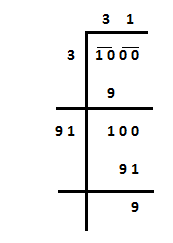Courses
Courses for Kids
Free study material
Free LIVE classes
MoreLIVE
Join Vedantu’s FREE Mastercalss

# Write the smallest four-digit number which is a perfect square.A. $1000$B. $1016$C. $1024$D. $1036$Verified
361.8k+ views
Hint: Find the square root of the smallest four-digit number. Use that information to get the answer.

We need to find the smallest four-digit number which is a perfect square. We know that 1000 is the smallest four-digit number. so, we’ll find the square root of that as follows:Now, from the above process what we have understood is, ${(31)^2} < 1000$. It’s obvious that ${(32)^2} > 1000$. Let cross check once.$32 \times 32 = 1024$. So, the smallest four-digit number is 1024.
Hence the correct option is C.

Note: For this kind of problem, it’s better to start the solution with the smallest/largest n-digit number then we can move further in the computation according to the given condition.
Last updated date: 16th Sep 2023
Total views: 361.8k
Views today: 8.61k